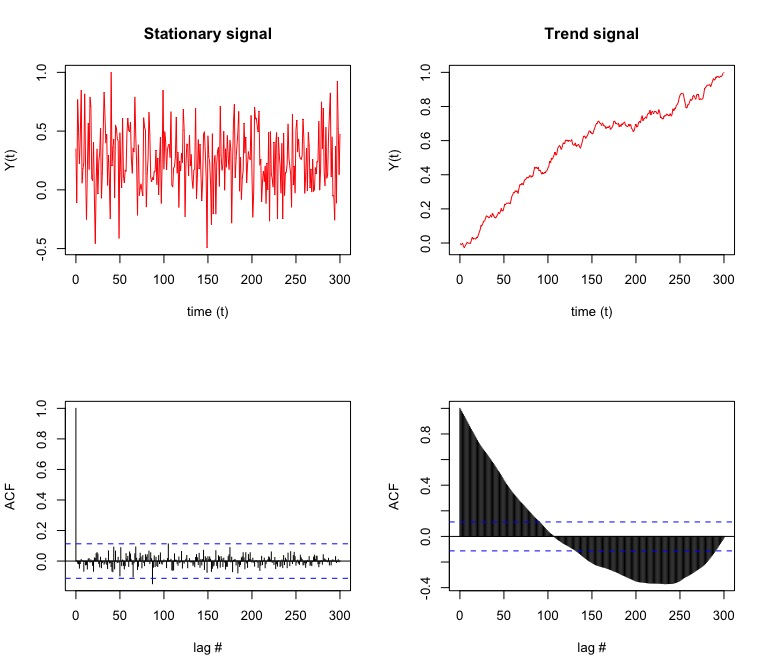# Stationarity Testing

When investigating a time series, one of the first things to check before building an ARIMA model is to check that the series is stationary. That is, it needs to be determined that the time series is constant in mean and variance are constant and not dependent on time.

Here, we will look at a couple methods for checking stationarity. If the time series is provided with seasonality, a trend, or a change point in the mean or variance, then the influences need to be removed or accounted for.

### Autocorrelation Function (ACF)

#### Identify if correlation at different time lags goes to 0

First, we can generate a couple example time series signals, y(t). One that we know is stationary (Guassian noise) and one with a trend (cummulative sum of Gaussian noise):

``````t = 0:300
y_stationary <- rnorm(length(t),mean=1,sd=1) # the stationary time series (ts)
y_trend      <- cumsum(rnorm(length(t),mean=1,sd=4))+t/100 # our ts with a trend
# lets normalize each for simplicity
y_stationary<- y_stationary/max(y_stationary)
y_trend      <- y_trend/max(y_trend) ``````

Second, we can check each for characteristics of stationarity by looking at the autocorrelation functions (ACF) of each signal. For a stationary signal, because we expect no dependence with time, we would expect the ACF to go to 0 for each time lag (τ). Lets visualize the signals and ACFs:

``````plot.new()
frame()
par(mfcol=c(2,2))
# the stationary signal and ACF
plot(t,y_stationary,
type='l',col='red',
xlab = "time (t)",
ylab = "Y(t)",
main = "Stationary signal")
acf(y_stationary,lag.max = length(y_stationary),
xlab = "lag #", ylab = 'ACF',main=' ')
# the trend signal and ACF
plot(t,y_trend,
type='l',col='red',
xlab = "time (t)",
ylab = "Y(t)",
main = "Trend signal")
acf(y_trend,lag.max = length(y_trend),
xlab = "lag #", ylab = 'ACF', main=' ')``````Notably, the stationary signal (top left) results in few significant lags that exceed the confidence interval of the ACF (blue dashed line, bottom left) . In comparison, the time series with a trend (top right) results in almost all lags exceeding the confidence interval of the ACF (bottom right). Qualitatively, we can see and conclude from the ACFs that the signal on the left is stationary (due to the lags that die out) while the signal on the right is not stationary (since later lags exceed the confidence interval).

### Ljung-Box test for independence

Quantitatively, we can also use built-in test for testing stationariy. First, the Ljung-Box test examines whether there is significant evidence for non-zero correlations at given lags (1-25 shown below), with the null hypothesis of independence in a given time series (a non-stationary signal will have a low p-value).

``````lag.length = 25
Box.test(y_stationary, lag=lag.length, type="Ljung-Box") # test stationary signal``````
``````##
##  Box-Ljung test
##
## data:  y_stationary
## X-squared = 8.0918, df = 25, p-value = 0.9994``````
``Box.test(y_trend,      lag=lag.length, type="Ljung-Box") # test nonstationary signal``
``````##
##  Box-Ljung test
##
## data:  y_trend
## X-squared = 5573.7, df = 25, p-value < 2.2e-16``````

### Augmented Dickey–Fuller (ADF) t-statistic test for unit root

Another test we can conduct is the Augmented Dickey–Fuller (ADF) t-statistic test to find if the series has a unit root (a series with a trend line will have a unit root and result in a large p-value).

``````options(warn=-1)
library(tseries)

``````##
##  Augmented Dickey-Fuller Test
##
## data:  y_stationary
## Dickey-Fuller = -6.8426, Lag order = 6, p-value = 0.01
## alternative hypothesis: stationary``````
``adf.test(y_trend)``
``````##
##  Augmented Dickey-Fuller Test
##
## data:  y_trend
## Dickey-Fuller = -2.161, Lag order = 6, p-value = 0.5084
## alternative hypothesis: stationary``````

### Kwiatkowski-Phillips-Schmidt-Shin (KPSS) for level or trend stationarity

Lastly, we can test if the time series is level or trend stationary using the Kwiatkowski-Phillips-Schmidt-Shin (KPSS) test. Here we will test the null hypothesis of trend stationarity (a low p-value will indicate a signal that is not trend stationary, has a unit root):

``kpss.test(y_stationary, null="Trend")``
``````##
##  KPSS Test for Trend Stationarity
##
## data:  y_stationary
## KPSS Trend = 0.057585, Truncation lag parameter = 4, p-value = 0.1``````
``kpss.test(y_trend, null="Trend")``
``````##
##  KPSS Test for Trend Stationarity
##
## data:  y_trend
## KPSS Trend = 1.2593, Truncation lag parameter = 4, p-value = 0.01``````